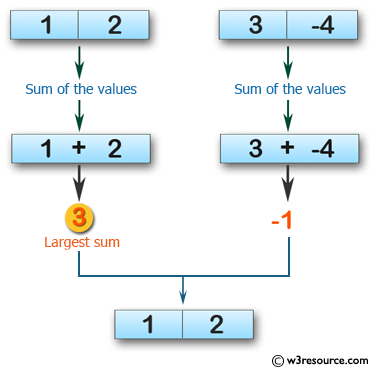﻿ Swift Array Programming Exercise: Compute the sum of the values of two given array of integers and each length 2. Find the array which has the largest sum and return the first array if the sum of two given arrays are equal - w3resource# Swift Array Programming Exercises: Compute the sum of the values of two given array of integers and each length 2. Find the array which has the largest sum and return the first array if the sum of two given arrays are equal

## Swift Array Programming: Exercise-16 with Solution

Write a Swift program to compute the sum of the values of two given array of integers and each length 2. Find the array which has the largest sum and return the first array if the sum of two given arrays are equal.

Pictorial Presentation:Sample Solution:

Swift Code:

``````func bigger_array(_ a: [Int], _ b: [Int]) -> [Int] {
if (a + a) >= (b + b)
{
return a
}
else
{
return b
}
}
print(bigger_array([1, 2], [3, -4]))
print(bigger_array([0, 1], [1, 2]))
print(bigger_array([6, 2], [4, 4]))
```
```

Sample Output:

```[1, 2]
[1, 2]
[6, 2]
```

Swift Programming Code Editor:

Improve this sample solution and post your code through Disqus

What is the difficulty level of this exercise?

﻿